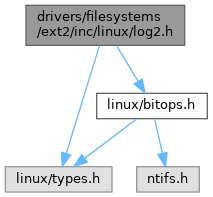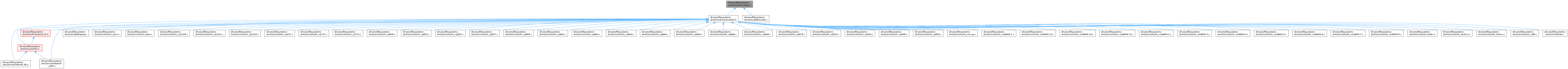ReactOS 0.4.15-dev-6069-g56a4501
log2.h File Reference
`#include <linux/types.h>`
`#include <linux/bitops.h>`
Include dependency graph for log2.h:This graph shows which files directly or indirectly include this file:Go to the source code of this file.

## Macros

#define ilog2(n)

#define roundup_pow_of_two(n)

#define rounddown_pow_of_two(n)

#define order_base_2(n)   ilog2(roundup_pow_of_two(n))

## Functions

int ____ilog2_NaN (void)

static __attribute__ ((const)) int __ilog2_u32(u32 n)

## ◆ ilog2

 #define ilog2 ( n )
Value:
( \
(sizeof(n) <= 4) ? \
__ilog2_u32(n) : \
__ilog2_u64(n) \
)
GLdouble n
Definition: glext.h:7729

ilog2 - log of base 2 of 32-bit or a 64-bit unsigned value
- parameter

constant-capable log of base 2 calculation

• this can be used to initialise global variables from constant data, hence the massive ternary operator construction

selects the appropriately-sized optimised version depending on sizeof(n)

Definition at line 84 of file log2.h.

## ◆ order_base_2

 #define order_base_2 ( n ) ilog2(roundup_pow_of_two(n))

order_base_2 - calculate the (rounded up) base 2 order of the argument
: parameter

The first few values calculated by this routine: ob2(0) = 0 ob2(1) = 0 ob2(2) = 1 ob2(3) = 2 ob2(4) = 2 ob2(5) = 3 ... and so on.

Definition at line 138 of file log2.h.

## ◆ rounddown_pow_of_two

 #define rounddown_pow_of_two ( n )
Value:
( \
__builtin_constant_p(n) ? ( \
(n == 1) ? 0 : \
(1UL << ilog2(n))) : \
__rounddown_pow_of_two(n) \
)
#define ilog2(n)
Definition: log2.h:84

rounddown_pow_of_two - round the given value down to nearest power of two
- parameter

round the given value down to the nearest power of two

• the result is undefined when n == 0
• this can be used to initialise global variables from constant data

Definition at line 116 of file log2.h.

## ◆ roundup_pow_of_two

 #define roundup_pow_of_two ( n )
Value:
( \
__builtin_constant_p(n) ? ( \
(n == 1) ? 1 : \
(1UL << (ilog2((n) - 1) + 1)) \
) : \
__roundup_pow_of_two(n) \
)

roundup_pow_of_two - round the given value up to nearest power of two
- parameter

round the given value up to the nearest power of two

• the result is undefined when n == 0
• this can be used to initialise global variables from constant data

Definition at line 99 of file log2.h.

## ◆ ____ilog2_NaN()

 int ____ilog2_NaN ( void )

## ◆ __attribute__()

 static __attribute__ ( (const) )
inlinestatic

Definition at line 30 of file log2.h.

32{
33 return fls(n) - 1;
34}
static int fls(int x)
Definition: bitops.h:157